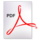# Lie Symmetry Techniques for Partial Differential Equations

## About the workshop

Lie symmetry methods can be useful for constructing exact solutions to differential equations. In this workshop, we will show how to determine the symmetry properties of linear and nonlinear partial differential equations, and how to exploit these properties to reduce the order of the governing equation. The workshop will focus on developing an understanding of the nuances in finding the symmetry classification of classes of differential equations. Simple Maple algorithms will be used to generate and solve determining equations. The symmetry determination package Dimsym (in Reduce) will be demonstrated. An overview of more generalised symmetry methods will also be discussed.

## Venue

The venue for the workshop is room SR202 in the SR building, at the University of Newcastle's Callaghan Campus.

## Workshop Overview

The workshop will cover

• one-parameter groups of transformations
• invariance of the governing differential equation
• prolongation formula
• algorithm for generating determining equations for classical Lie point symmetries
• symmetry properties of the linear diffusion equation (Maple)
• symmetry classification of the nonlinear diffusion equation (Maple)
• symmetry classification of the nonlinear convection-diffusion (Maple)
• symmetry classification of the nonlinear diffusion equation (Dimsym)
• nonclassical symmetry methods
• other generalised symmetry methods

## Registration

Registration is now open through Eventbrite. Note that while there is no cost to attend, you will need to register to ensure a place at the workshop.

## Prerequisites

• Introduction to PDEs (a second year course on PDEs)
• Ability to write basic Maple (or equivalent) code will be an advantage. These skills will be developed during the workshop.

## Resources

These will be distributed to participants prior to the workshop.

• Maureen Edwards, One Parameter Groups
• Sample Maple and Dimsym code
• Installation instructions: Dimsym and Reduce

## Technical requirements

Workshop participants should bring a laptop with Maple, and with Reduce and Dimsym installed.Click here to download the instructions for installing these packages (308 KB PDF file).

## Schedule### Thursday 9th May

We will discuss one-parameter groups of transformations. We will work though a number of examples, demonstrating how to obtain the determining equations and how to solve them to find the relevant symmetry group. Examples include:

• the linear heat equation
For this example, we will find the well known symmetry group by hand, by using Maple (where some interaction is required), and by using dimsym/Reduce (which requires little interaction)
• the nonlinear heat equation with $D(u) = u^n$
For this example, we will find the symmetry group using both Maple and dimsym/Reduce. An additional symmetry is found for $n = -\frac{4}{3}$.
• the nonlinear heat equation with general $D(u)$
In this case, we will examine the determining equations using Maple and dimsym/Reduce, and see how the special case $D(u) = u^n$ arises.

### Friday 10th May

On the second day we will discuss how to use the symmetry groups found on the first day. In particular, we will look at examples including:

• the linear heat equation — including the Boltzmann reduction, travelling waves, and more
• the nonlinear heat equation with $D(u) = u^n$ — including travelling waves and more

We will also give a brief introduction to generalised symmetries, including nonlocal symmetries, and nonclassical symmetries.

## Biographies

Dr Maureen Edwards

Maureen is a Senior Lecturer in applied mathematics at the University of Wollongong. Her research interests include the use of symmetry analysis for the construction of exact solutions of nonlinear differential equations, motivated by physical phenomena such as biological invasion and population dynamics.

Dr Bronwyn Hajek

Bronwyn is a Senior Lecturer in applied mathematics at the University of South Australia. Her research interests lie in the use of symmetry techniques to construct analytic solutions of nonlinear reaction-diffusion equations, particularly with application to problems in mathematical biology. She has also worked on problems in viscous flow and physical chemistry.